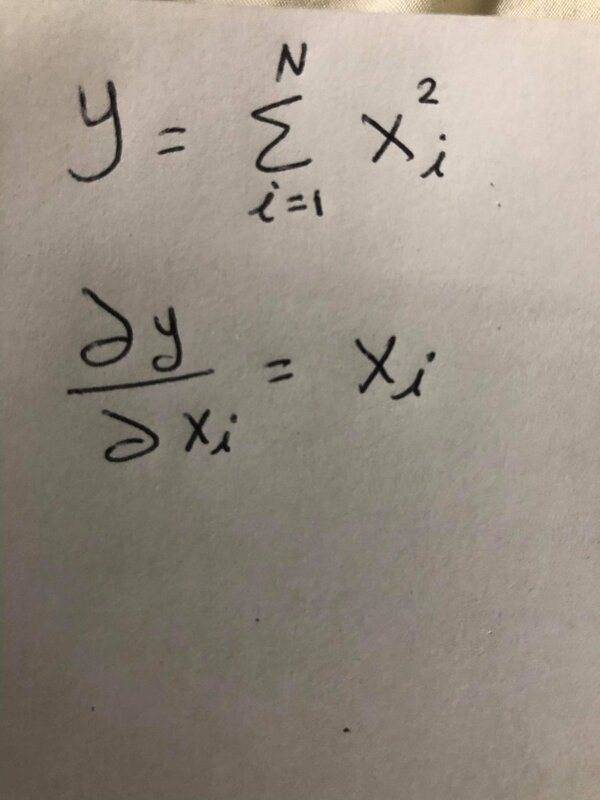# Partial Derivative of a Sum

• I
• Ryan187
No. ## i ## is just one specific index, an arbitrary one, but a specific one. That's why we have to distinguish this index from the summation index which runs over all. One of them matches ##j=i## whereas all others do not: ##j\neq i##. The former yields ##2x_i##, the latter yields the zeros.Got it now! Thanks, guys, you're amazing!f

#### Ryan187

Why the summation of the following function will be canceled out when we take the partial derivative with respect to the x_i?
Notice that x_i is the sub of (i), which is the same lower limit of the summation! Can someone, please explain in details?The ##x_i## are, I assume, independent variables. If ##i \ne j## then ##\frac{\partial x_j}{\partial x_i} = 0##.

Note that the answer should be ##2x_i##.

Thank you PeroK! Even if x_i is an independent variable. why the summation is canceled out? can you explain the derivative in detail, please? and you're right the answer is 2x_i not x_i

Thank you PeroK! Even if x_i is an independent variable. why the summation is canceled out? can you explain the derivative in detail, please? and you're right the answer is 2x_i not x_i
The summation is not needed because most terms in the sum are zero.

•Ryan187 and etotheipi
PS it might help is you use a different dummy variable for the definition of ##y##. Use ##j = 1## to ##n## instead of ##i##, for example.

@Ryan187 if you replace the free index ##i## with a concrete number, can you for instance evaluate$$\frac{\partial}{\partial x_3} \sum_{k=1}^5 ({x_k}^2)= \frac{\partial}{\partial x_3} ({x_1}^2 + {x_2}^2 + {x_3}^2 + {x_4}^2 + {x_5}^2)$$assuming the ##x_i## are independent variables?

•Ryan187 and Mark44
explain
Differentiation is a linear function: ##\dfrac{\partial}{\partial x_j}(\alpha \cdot f(x)+\beta\cdot g(x))=\alpha\cdot \dfrac{\partial}{\partial x_j}f(x)+\beta\cdot \dfrac{\partial}{\partial x_j} g(x)##.

Here we only have a longer sum: ##x_1^2+x_2^2+\ldots+x_N^2##

•Ryan187 and etotheipi
@Ryan187 if you replace the free index ##i## with a concrete number, can you for instance evaluate$$\frac{\partial}{\partial x_3} \sum_{k=1}^5 ({x_k}^2)= \frac{\partial}{\partial x_3} ({x_1}^2 + {x_2}^2 + {x_3}^2 + {x_4}^2 + {x_5}^2)$$assuming the ##x_i## are independent variables?
This is the key -- expanding the sum, and then differentiating with respect to one of the terms in the sum.

•Ryan187 and etotheipi
So, in this case, I am kind of differentiating over one term that's why the sum will be canceled out?

So, in this case, I am kind of dif\displaystyle{}ferentiating over one term that's why the sum will be canceled out?
No. You differentiate all terms. ##\displaystyle{\dfrac{\partial}{\partial x_i}\left(\sum_{j=1}^N x_j^2\right) = \sum_{j=1}^N \dfrac{\partial}{\partial x_i} (x_j^2)}##

•Ryan187 and etotheipi
I'll use my example again\begin{align*}\frac{\partial}{\partial x_3} \sum_{k=1}^5 ({x_k}^2)&= \frac{\partial}{\partial x_3} ({x_1}^2 + {x_2}^2 + {x_3}^2 + {x_4}^2 + {x_5}^2)\\ \\&= \frac{\partial}{\partial x_3}({x_1}^2) + \frac{\partial}{\partial x_3}({x_2}^2) + \frac{\partial}{\partial x_3}({x_3}^2)+ \frac{\partial}{\partial x_3}({x_4}^2)+\frac{\partial}{\partial x_3}({x_5}^2) \\ \\ &= 0 + 0 + \frac{\partial}{\partial x_3}({x_3}^2) + 0 + 0\end{align*}I see I've been beaten to it by @fresh_42•Ryan187
I see I've been beaten to it by @fresh_42I have these shortcuts on the keyboard:

\displaystyle{}
\dfrac{}{}
\left(\right)
\partial••jim mcnamara and etotheipi
but in this case, we derive with respect to x3 (i=3) which is one term, but the original derivative is with respect to (x_i) which is the whole i's values?!

but in this case, we derive with respect to x3 (i=3) which is one term, but the original derivative is with respect to (x_i) which is the whole i's values?!
No. ## i ## is just one specific index, an arbitrary one, but a specific one. That's why we have to distinguish this index from the summation index which runs over all. One of them matches ##j=i## whereas all others do not: ##j\neq i##. The former yields ##2x_i##, the latter yields the zeros.

•Ryan187
Got it now! Thanks, guys, you're amazing! It is my first thread to this community!

Last edited:
•etotheipi